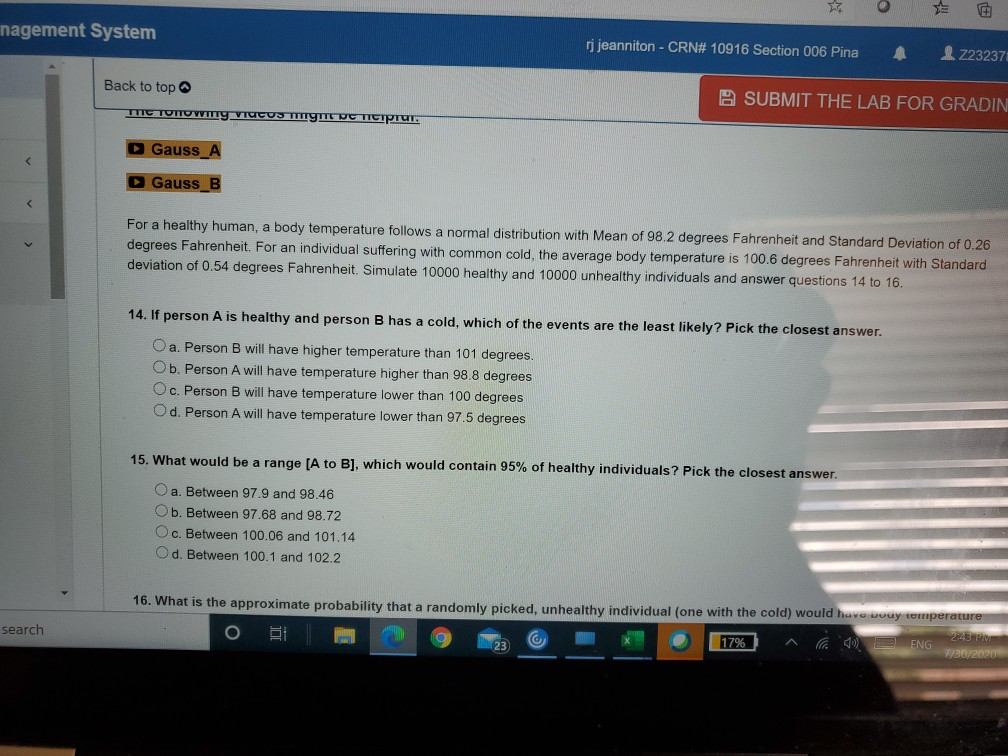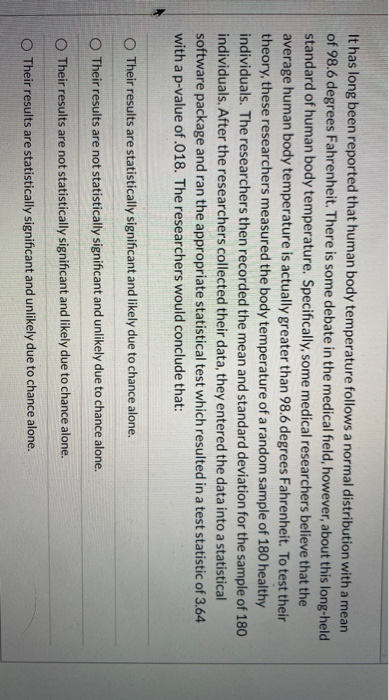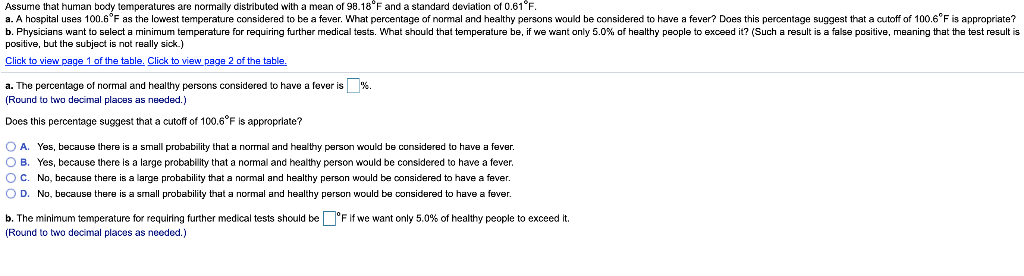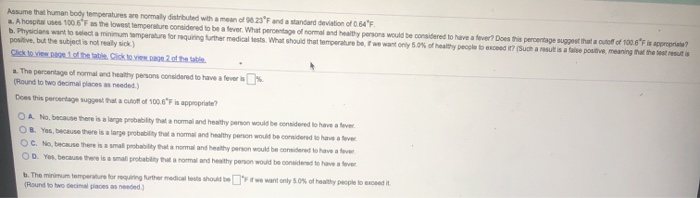Question

# For a healthy human, a body temperature follows a normal distribution with Mean of 98.2 degrees...

For a healthy human, a body temperature follows a normal distribution with Mean of 98.2 degrees Fahrenheit and Standard Deviation of 0.26 degrees Fahrenheit. For an individual suffering with common cold, the average body temperature is 100.6 degrees Fahrenheit with Standard deviation of 0.54 degrees Fahrenheit. Simulate 10000 healthy and 10000 unhealthy individuals and answer questions 14 to 16.

14. If person A is healthy and person B has a cold, which of the events are the least likely? Pick the closest answer.

• a. Person B will have higher temperature than 101 degrees.
• b. Person A will have temperature higher than 98.8 degrees
• c. Person B will have temperature lower than 100 degrees
• d. Person A will have temperature lower than 97.5 degrees

15. What would be a range [A to B], which would contain 95% of healthy individuals? Pick the closest answer.

• a. Between 97.9 and 98.46
• b. Between 97.68 and 98.72
• c. Between 100.06 and 101.14
• d. Between 100.1 and 102.2

16. What is the approximate probability that a randomly picked, unhealthy individual (one with the cold) would have body temperature below 100 degrees Fahrenheit? Pick the closest answer.

14)

• d. Person A will have temperature lower than 97.5 degrees

15)

• b. Between 97.68 and 98.72

16)

#### Earn Coins

Coins can be redeemed for fabulous gifts.

Similar Homework Help Questions
• ### TE nagement System rj jeanniton - CRN# 10916 Section 006 Pina 223237 Back to top 2...TE nagement System rj jeanniton - CRN# 10916 Section 006 Pina 223237 Back to top 2 SUBMIT THE LAB FOR GRADIN TII TUTTU Vity VUCUS TIITEV TICIPIUT. Gauss A Gauss_B For a healthy human, a body temperature follows a normal distribution with Mean of 98.2 degrees Fahrenheit and Standard Deviation of 0.26 degrees Fahrenheit. For an individual suffering with common cold, the average body temperature is 100.6 degrees Fahrenheit with Standard deviation of 0.54 degrees Fahrenheit. Simulate 10000 healthy and...

• ### It has long been reported that human body temperature follows a normal distribution with a mean...It has long been reported that human body temperature follows a normal distribution with a mean of 98.6 degrees Fahrenheit. There is some debate in the medical field, however, about this long-held standard of human body temperature. Specifically, some medical researchers believe that the average human body temperature is actually greater than 98.6 degrees Fahrenheit. To test their theory, these researchers measured the body temperature of a random sample of 180 healthy individuals. The researchers then recorded the mean and...

• ### The body temperatures of a group of healthy adults have a bell-shaped distribution with a mean of 98.33°F and a standard deviation of 0.65°F.

The body temperatures of a group of healthy adults have a bell-shaped distribution with a mean of 98.33°F and a standard deviation of 0.65°F. Using the empirical rule, find each approximate percentage below. a. What is the approximate percentage of healthy adults with body temperatures within 3 standard deviations of the mean, or between 96.38°F and 100.28°F? b. What is the approximate percentage of healthy adults with body temperatures between 97.68 °F and 98.98 °F? a. Approximately _______ % of healthy adults in...

• ### Suppose the body temperatures in the population of all healthy adults follow a normal distribution with...

Suppose the body temperatures in the population of all healthy adults follow a normal distribution with a mean of 98.6 degrees F and a standard deviation of 0.7 degrees F. Would it be unusual for a healthy adult to have a temperature of 100.5 degrees F? A. Yes. B. No. C. I have no idea. D. None of the above.

• ### Assume that human body temperatures are normally distributed with a mea of 98.18°F and a standard deviation of 0.61 F a. A hospital uses 100.6 F as the lowest temperature considered to be a fever. Wh...Assume that human body temperatures are normally distributed with a mea of 98.18°F and a standard deviation of 0.61 F a. A hospital uses 100.6 F as the lowest temperature considered to be a fever. What percentage of nomal and healthy persons would be considered to have a fever? Does this percentage suggest that a cutoff of 100.6 F is appropriate? b. Physicians want to select a minimum temperature for requiring further medical tests. What should that temperature be, if...

• ### In the 19th century, it was determined by experimentation that human body temperature is normally distributed...

In the 19th century, it was determined by experimentation that human body temperature is normally distributed N(98.6, 0.6) (in Fahrenheit F). Recently a new study has proposed that this should be modified to indicate that human body temperature is approximately normally distributed N(98.2,0.7) (F). a. Which of these two have a larger range of temperatures that represent 95% of the most common body temperatures? Provide an analysis that supports your conclusion. b. Over the course of 20 years an individual...

• ### What is normal, when it comes to people's body temperatures? A random sample of 130 human body temperatures had a mean of 98.15° and a standard deviation of 0.74°. Does the data indicate that the average body temperature for healthy humans deviates from 9

(a)Test using the p-value approach with ? = 0.05.State the null and alternative hypothesis.H0: ? < 98.6 versus Ha: ? > 98.6H0: ? = 98.6 versus Ha: ? > 98.6    H0: ? = 98.6 versus Ha: ? < 98.6H0: ? = 98.6 versus Ha: ? ≠ 98.6H0: ? ≠ 98.6 versus Ha: ? = 98.6Find the test statistic and the p-value. (Round your test statistic to two decimal places and your p-value to four decimal places.)z=p-value=State your conclusion.The p-value is greater than alpha so H0 is not rejected. There is insufficient evidence to indicate that the average body temperature for healthy humans deviates from 98.6°.The p-value is less than alpha so H0 is rejected. There is sufficient evidence to...

• ### What is normal, when it comes to people's body temperatures? A random sample of 130 human body temperatures had a mean of 98.15° and a standard deviation of 0.74°. Does the data indicate that the average body temperature for healthy humans deviates from 9

Test using the p-value approach with ? = 0.05.State the null and alternative hypothesis.H0: ? < 98.6 versus Ha: ? > 98.6H0: ? = 98.6 versus Ha: ? > 98.6    H0: ? = 98.6 versus Ha: ? < 98.6H0: ? = 98.6 versus Ha: ? ≠ 98.6H0: ? ≠ 98.6 versus Ha: ? = 98.6Find the test statistic and the p-value. (Round your test statistic to two decimal places and your p-value to four decimal places.)z=p-value=State your conclusion.The p-value is greater than alpha so H0 is not rejected. There is insufficient evidence to indicate that the average body temperature for healthy humans deviates from 98.6°.The p-value is less than alpha so H0 is rejected. There is sufficient evidence to...

• ### Assume that human body temperatures are normal distributed with mean s and a standard deviation of...Assume that human body temperatures are normal distributed with mean s and a standard deviation of 2. Ahol 1006F the lowest temperature considered to be a fever. What percentage of normal and healthy persons would be considered to have a ver? Does this percentage suggest a cut of 100.6Fir ? b. Physicians want to let a minimum smperature forquiring the medical What should hamperature bewewantony 5.0% of healthy people be c aus e positive meaning that the best result is...

• ### There is a linear relationship between the high temperature of the day (measured in degrees Fahrenheit)...

There is a linear relationship between the high temperature of the day (measured in degrees Fahrenheit) and the number of ice cream cones sold at Disneyland. Consider the correlation r between the high temperature of the day and the number of ice cream cones sold. Which one of the following statements is true about this correlation? A r in this example would have the units of “degrees Fahrenheit per cone.” B If higher temperatures are associated with fewer ice cream...